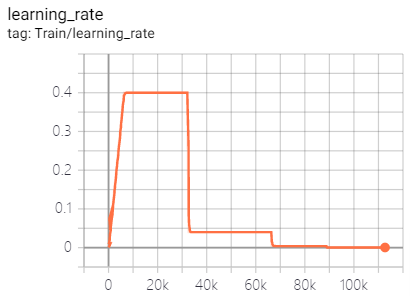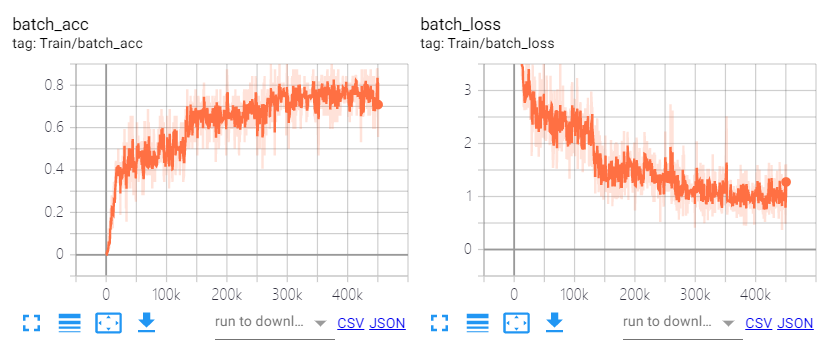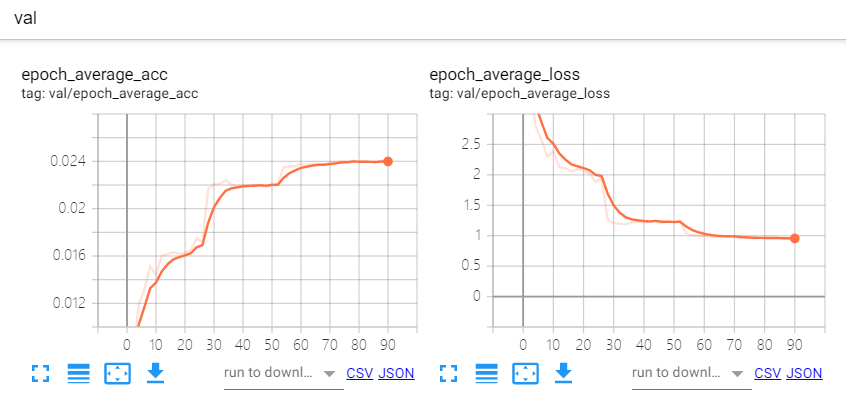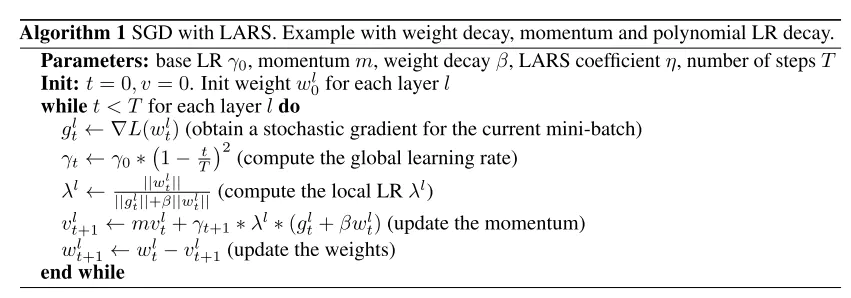# 提升分类模型acc(一)：BatchSize&LARS¶

【GiantPandaCV导读】在使用大的bs训练情况下，会对精度有一定程度的损失，本文探讨了训练的bs大小对精度的影响，同时探究LARS是否可以有效的提升精度。

## 二、实验配置¶

• 模型：ResNet50
• 数据：ImageNet1k
• 环境：8xV100

## 三、BatchSize对精度的影响¶

• lr调整曲线如下：• 训练曲线如下:• 验证曲线如下:ResNet50 90 0.1 256 randomcropresize,randomflip 76.422%
ResNet50 90 0.4 1024 randomcropresize,randomflip 76.228%
ResNet50 90 0.8 2048 randomcropresize,randomflip 76.132%
ResNet50 90 1.6 4096 randomcropresize,randomflip 75.75%

### 1. 理论分析¶

LARS的出发点则是各个层的更新参数使用的学习率应该根据自己的情况有所调整，而不是所有层使用相同的学习率，也就是每层有自己的local lr，所以有：

\lambda^{l} = \eta * \frac{||w^{l}||}{||\nabla L(w^{l})|| + \beta * ||w^{l}||}

\nabla w_{t}^{l} = \gamma * \lambda^{l} * \nabla L(w_{t}^{l})LARS代码如下：

class LARC(object):
def __init__(self, optimizer, trust_coefficient=0.02, clip=True, eps=1e-8):
self.optim = optimizer
self.trust_coefficient = trust_coefficient
self.eps = eps
self.clip = clip

def step(self):
weight_decays = []
for group in self.optim.param_groups:
# absorb weight decay control from optimizer
weight_decay = group['weight_decay'] if 'weight_decay' in group else 0
weight_decays.append(weight_decay)
group['weight_decay'] = 0
for p in group['params']:
continue
param_norm = torch.norm(p.data)

if param_norm != 0 and grad_norm != 0:
# calculate adaptive lr + weight decay
adaptive_lr = self.trust_coefficient * (param_norm) / (
grad_norm + param_norm * weight_decay + self.eps)

# clip learning rate for LARC
if self.clip:
# calculation of adaptive_lr so that when multiplied by lr it equals min(adaptive_lr, lr)

self.optim.step()
# return weight decay control to optimizer
for i, group in enumerate(self.optim.param_groups):
group['weight_decay'] = weight_decays[i]


### 2. 实验结论¶

ResNet50 90 0.4 1024 randomcropresize,randomflip 75.146% 1e-3
ResNet50 90 0.8 2048 randomcropresize,randomflip 73.946% 1e-3
ResNet50 90 1.6 4096 randomcropresize,randomflip 72.396% 1e-3
ResNet50 90 0.4 1024 randomcropresize,randomflip 76.234% 2e-2
ResNet50 90 0.8 2048 randomcropresize,randomflip 75.898% 2e-2
ResNet50 90 1.6 4096 randomcropresize,randomflip 75.842% 2e-2

LARS一定程度上可以提升精度，但是强依赖超参，还是需要细致的调参训练。

## 四、结论¶

• 8卡进行分布式训练，使用1k的bs可以很好的平衡acc&speed。
• LARS一定程度上可以提升精度，但是需要调参，做业务可以不用考虑，刷点的话要好好训练。